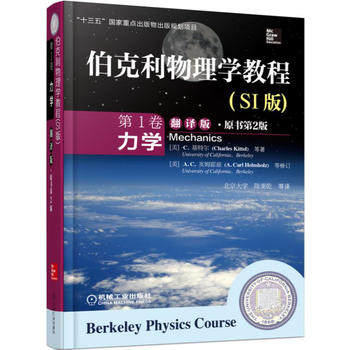## 质心参考系中处理两个粒子的弹性碰撞《伯克利物理学教程·力学》翻译版封面\begin{equation} M_1v_1=M_1v'_1\cos\theta_1+M_2v'_2\cos\theta_2 \label{momentumconsx} \end{equation}

\begin{equation} 0=M_1v'_1\sin\theta_1+M_2v'_2\sin\theta_2 \label{momentumconsy} \end{equation}

\begin{equation} \frac{1}{2}M_1v_1^2=\frac{1}{2}M_1{v'}_1^2 + \frac{1}{2}M_2{v'}_2^2 \label{energycons} \end{equation}

- 阅读剩余部分 -

## 水与水分子## 波的动图## 推导惯性系之间的坐标变换- 阅读剩余部分 -

## 由伽利略不变性推导动量守恒定律- 阅读剩余部分 -

## 转动小球的自由下落与地面的碰撞后的反跳- 阅读剩余部分 -

## 专家谈：锻炼能增寿几何？- 阅读剩余部分 -

## 劈尖干涉测量金属丝杨氏模量

$$E=\frac{F/S}{\Delta l/l_0}\tag{1}$$- 阅读剩余部分 -

## 大球和小球摞在一起时的反跳- 阅读剩余部分 -